## Plot the Impulse Response Function of Conditional Mean Model

This topic presents several examples that show how to plot and return the impulse response function (IRF) of univariate autoregressive moving average (ARMA) models. The examples also show how to interact with the plots.

The Econometrics Toolbox™ functions `impulse` and `armairf` use the same method to compute the IRF of a univariate conditional mean model, by default. However, the functions have differences, as described in this table.

FunctionDescriptionRequired InputNotes
`impulse`Plots (computes) IRF of an ARIMA model specified by an `arima` model objectFully specified `arima` model object, such as a model returned by `estimate`

• Applies a unit shock at time 0 (ε0 = 1)

• Plots a stem plot

• Well suited for `arima` model object workflows, particularly seasonal or integrated models

`armairf`Plots (computes) IRF of an ARIMA model specified by overall AR and MA lag operator polynomialsArrays containing the overall AR and MA lag operator polynomial coefficients, or `LagOp` lag operator polynomial objects representing the overall AR and MA components

• Applies a one-standard-deviation shock at time 0 (ε0 = σ)

• Plots a time series plot

• Well suited for plot manipulation

• Supports multivariate linear time series models

### IRF of Moving Average Model

This example shows how to plot and return the IRF of a pure MA model by using `impulse` and `armairf`. The example also shows how to change the color of the plotted IRFs.

The equation for an MA(q) model is

`${y}_{t}=\mu +\theta \left(L\right){\epsilon }_{t},$`

where $\theta \left(L\right)$ is a q-degree MA lag operator polynomial, $\left(1+{\theta }_{1}L+\dots +{\theta }_{q}{L}^{q}\right).$

The IRF for an MA model is the sequence of MA coefficients $1,{\theta }_{1},\dots ,{\theta }_{q}.$

Plot IRF Using `impulse`

Create a zero-mean MA(3) model with coefficients ${\theta }_{1}=0.8$, ${\theta }_{2}=0.5$, and ${\theta }_{3}=-0.1,$ and an innovation variance of 1.

```ma = [0.8 0.5 -0.1]; Mdl = arima('Constant',0,'MA',ma,'Variance',1);```

`Mdl` is a fully specified `arima` model object representing the MA(3) model.

Plot the IRF of the MA(3) model.

`impulse(Mdl)``impulse` returns a stem plot containing 1 at period 0, followed by the values of the MA coefficients at their lags.

For an MA model, the impulse response function stops after q periods. In this example, the last nonzero coefficient is at lag q = 3.

Return the IRF by calling `impulse` and specifying an output argument.

```periods = (0:3)'; dm = impulse(Mdl); IRF = table(periods,dm)```
```IRF=4×2 table periods dm _______ ____ 0 1 1 0.8 2 0.5 3 -0.1 ```

To change aspects of the stem plot, you must set values of its properties. The stem plot handle is in the `Children` property of the plot axes handle.

Extract the stem plot handle from the current axes handle.

```h = gca; hstem = h.Children;```

Change the color of the stem plot to red by using the RGB color value.

`hstem.Color = [1 0 0]; `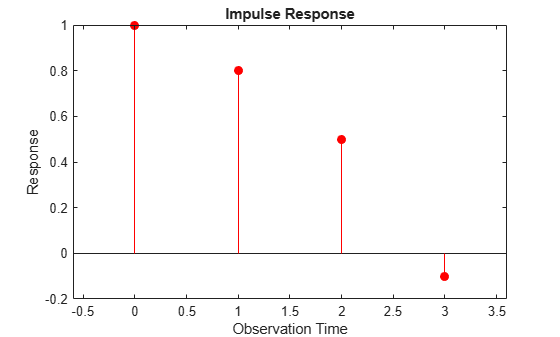Plot IRF Using `armairf`

Plot the IRF of the MA(3) model by passing `ma` as the MA coefficients (second input). Specify an empty array for the AR polynomial coefficients (first input). Return the IRF and plot handle.

`[dm,h] = armairf([],ma);`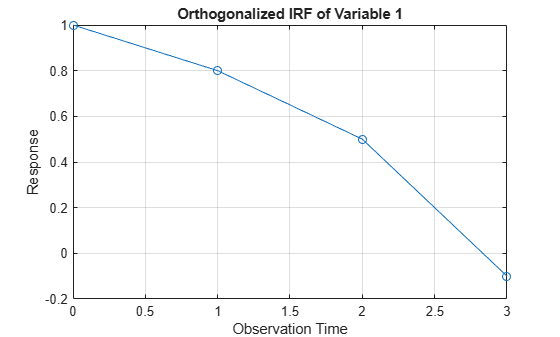`table(periods,dm)`
```ans=4×2 table periods dm _______ ____ 0 1 1 0.8 2 0.5 3 -0.1 ```

Unlike `impulse`, `armairf` returns a time series plot.

Change the color of the plot line to red.

`h.Color = [1 0 0];`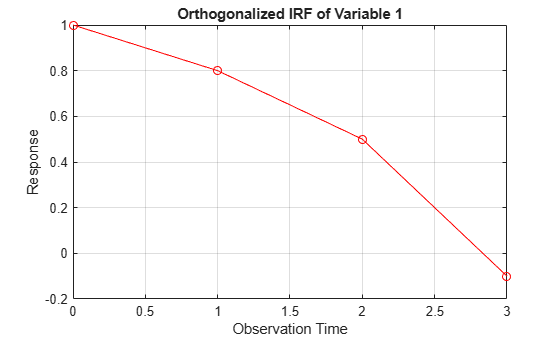### IRF of Autoregressive Model

This example shows how to plot the IRF of an AR model by using `impulse` and `armairf`. Also, the example shows how changes to the innovation variance affect the IRF.

The equation of an AR(p) model is

`${y}_{t}=c+\varphi \left(L{\right)}^{-1}{\epsilon }_{t},$`

where $\varphi \left(L\right)$ is the $p$-degree AR lag operator polynomial $\left(1-{\varphi }_{1}L-\dots -{\varphi }_{p}{L}^{p}\right)$.

An AR process is stationary when the AR lag operator polynomial is stable, which means all its roots lie outside the unit circle. In this case, the infinite-degree inverse polynomial $\psi \left(L\right)=\varphi \left(L{\right)}^{-1}$ has absolutely summable coefficients, and the IRF decays to zero.

Plot IRF Using `impulse`

Create an AR(2) model with coefficients ${\varphi }_{1}=0.5$ and ${\varphi }_{2}=-0.75$, a model constant of 0.5, and an innovation variance of 1.

```ar = [0.5 -0.75]; Mdl = arima('Constant',0.5,'AR',ar,'Variance',1);```

Plot the IRF of the AR(2) model for 31 periods, from periods 0 through 30.

```numObs = 31; impulse(Mdl,numObs)```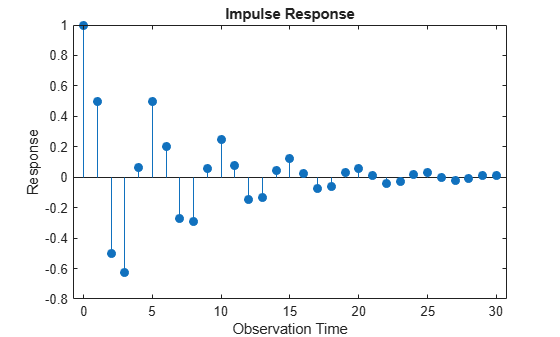The IRF decays in a sinusoidal pattern.

Increase the constant to 100, and then plot the IRF of the adjusted AR(2) model.

```Mdl.Constant = 100; impulse(Mdl,numObs)```Because deterministic components are not present in the IRF, it is unaffected by the increased constant.

Decrease the innovation variance to `1e-5`, and then plot the IRF of the adjusted AR(2) model.

```Mdl.Variance = 1e-5; impulse(Mdl,numObs)```Because `impulse` always applies a unit shock to the innovation of the system, the IRF is unaffected by the decreased innovation variance.

Plot IRF Using `armairf`

Plot the IRF of the original AR(2) model by passing `ar` as the AR coefficients (first input). Specify an empty array for the MA polynomial coefficients (second input). Specify 31 periods.

`armairf(ar,[],'NumObs',numObs)`Plot the IRF specifying an innovation variance of `1e-5`.

`armairf(ar,[],'NumObs',numObs,'InnovCov',1e-5);`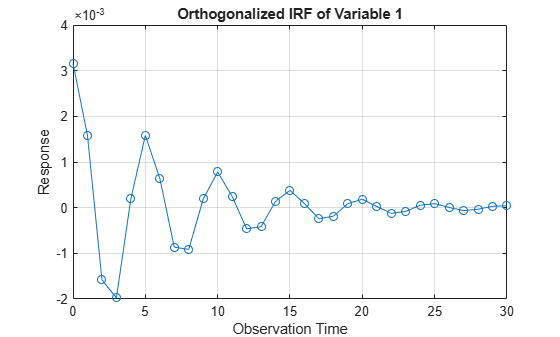Because `armairf` applies a one-standard-deviation innovation shock to the system, the scale of the IRF is smaller in this case.

### IRF of ARMA Model

This example shows how to plot the IRF of an ARMA model by using `impulse` and `armairf`.

The equation of an ARMA(p,q) model is

`${y}_{t}=c+\varphi \left(L{\right)}^{-1}\theta \left(L\right){\epsilon }_{t},$`

where:

• $\varphi \left(L\right)$ is the p-degree AR lag operator polynomial $\left(1-{\varphi }_{1}L-\dots -{\varphi }_{p}{L}^{p}\right)$.

• $\theta \left(L\right)$ is the q-degree MA lag operator polynomial $\left(1+{\theta }_{1}L+\dots +{\theta }_{q}{L}^{q}\right)$.

An ARMA process is stationary when the AR lag operator polynomial is stable, which means all its roots lie outside the unit circle. In this case, the infinite-degree inverse polynomial $\psi \left(L\right)=\varphi \left(L{\right)}^{-1}\theta \left(L\right)$ has absolutely summable coefficients, and the IRF decays to zero.

Plot IRF Using `impluse`

Create an ARMA(2,1) model with coefficients ${\varphi }_{1}=0.6$, ${\varphi }_{2}=-0.3$, and ${\theta }_{1}=0.4$, a model constant of 0, and an innovation variance of 1.

```ar = [0.6 -0.3]; ma = 0.4; Mdl = arima('AR',ar,'MA',ma,'Constant',0,'Variance',1);```

Plot the IRF of the ARMA(2,1) model for 11 periods, from periods 0 through 10.

```numObs = 11; impulse(Mdl,numObs)```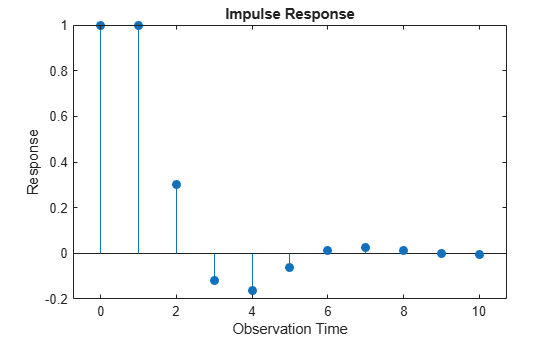The IRF decays in a sinusoidal pattern.

Plot IRF Using `armairf`

Plot the IRF of the ARMA(2,1) model by passing `ar` as the AR coefficients (first input) and `ma` as the MA coefficients (second input). Specify 11 periods.

`armairf(ar,ma,'NumObs',numObs)`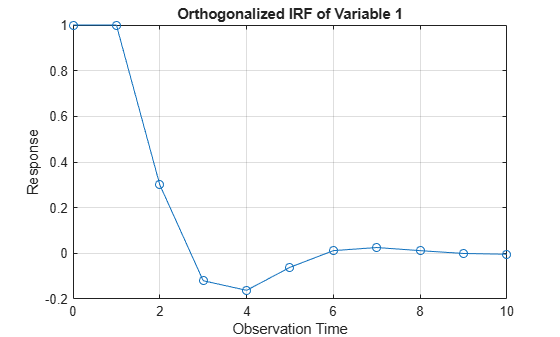### IRF of Seasonal AR Model

This example shows how to plot and return the IRF of a seasonal AR model by using `impulse` and `armairf`. Also, the example shows how to prepare `LagOp` lag operator polynomials as inputs to `armairf`.

The equation of an SAR$\left(\mathit{p},0,0\right)×\left({\mathit{p}}_{\mathit{s}},0,0{\right)}_{\mathit{s}}$ model is

${y}_{t}=c+\Phi \left(L{\right)}^{-1}\varphi \left(L{\right)}^{-1}{\epsilon }_{t}$,

where:

• $\varphi \left(\mathit{L}\right)$ is the $\mathit{p}$-degree AR lag operator polynomial $1-{\varphi }_{1}\mathit{L}-...-{\varphi }_{\mathit{p}}{\mathit{L}}^{\mathit{p}}$.

• $\Phi \left(\mathit{L}\right)$ is the ${\mathit{p}}_{\mathit{s}}$-degree seasonal AR lag operator polynomial $1-{\Phi }_{{\mathit{p}}_{1}}{\mathit{L}}^{{\mathit{p}}_{1}}-...-{\Phi }_{{\mathit{p}}_{\mathit{s}}}{\mathit{L}}^{{\mathit{p}}_{\mathit{s}}}$.

Like a pure AR process, an SAR process is stationary when the product $\varphi \left(\mathit{L}\right)\Phi \left(\mathit{L}\right)$ is stable. In this case, the infinite-degree inverse polynomial $\psi \left(L\right)=\Phi \left(L{\right)}^{-1}\varphi \left(L{\right)}^{-1}$ has absolutely summable coefficients, and the IRF decays to zero.

Plot IRF Using `impulse`

Create a quarterly SAR$\left(1,0,0\right)×{\left(4,0,0\right)}_{4}$ model with coefficients ${\varphi }_{1}=0.5$ and ${\Phi }_{4}=-0.4$, a model constant of 0, and an innovation variance of 1.

```ar = 0.5; sar = -0.4; sarlags = 4; Mdl = arima('AR',ar,'SAR',sar,'SARLags',sarlags,... 'Constant',0,'Variance',1)```
```Mdl = arima with properties: Description: "ARIMA(1,0,0) Model with Seasonal AR(4) (Gaussian Distribution)" SeriesName: "Y" Distribution: Name = "Gaussian" P: 5 D: 0 Q: 0 Constant: 0 AR: {0.5} at lag  SAR: {-0.4} at lag  MA: {} SMA: {} Seasonality: 0 Beta: [1×0] Variance: 1 ```

Plot the IRF of the SAR model for 17 quarters, from quarters 0 through 16.

```numObs = 17; impulse(Mdl,numObs)```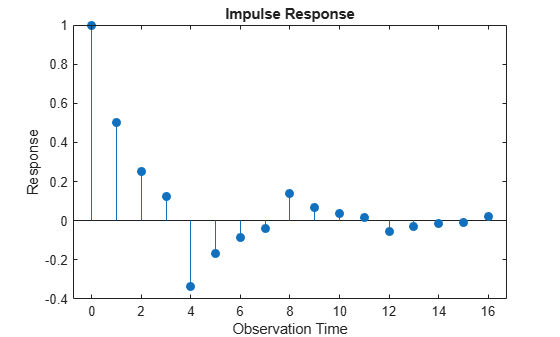The IRF decays in a sinusoidal pattern.

Return the IRF.

`irfIMPULSE = impulse(Mdl,numObs);`

Plot IRF Using `armirf`

`armairf` accepts one overall AR polynomial. Therefore, you must multiply all AR and differencing lag operator polynomials present in the model before calling `armairf`.

Create lag operator polynomials for the AR and SAR polynomials. For each polynomial:

• Include the lag 0 term, which has a coefficient of 1.

• Negate the coefficients to express the polynomials in lag operator notation, with all AR polynomials on the left side of the equation.

`ARLOP = LagOp([1 -ar],'Lags',[0 1])`
```ARLOP = 1-D Lag Operator Polynomial: ----------------------------- Coefficients: [1 -0.5] Lags: [0 1] Degree: 1 Dimension: 1 ```
`MALOP = LagOp([1 -sar],'Lags',[0 sarlags])`
```MALOP = 1-D Lag Operator Polynomial: ----------------------------- Coefficients: [1 0.4] Lags: [0 4] Degree: 4 Dimension: 1 ```

Multiply the polynomials.

`ARProdLOP = ARLOP*MALOP`
```ARProdLOP = 1-D Lag Operator Polynomial: ----------------------------- Coefficients: [1 -0.5 0.4 -0.2] Lags: [0 1 4 5] Degree: 5 Dimension: 1 ```

`ARProdLOP` is a `LagOp` object representing the product of the AR and SAR polynomials of the SAR model.

Plot and return the IRF by passing `ARProdLOP` as the AR polynomial (first input). Specify an empty array for the MA polynomial (second input). To plot the IRF, also return the plot handle.

`[irfARMAIRF,h] = armairf(ARProdLOP,[],'NumObs',numObs);`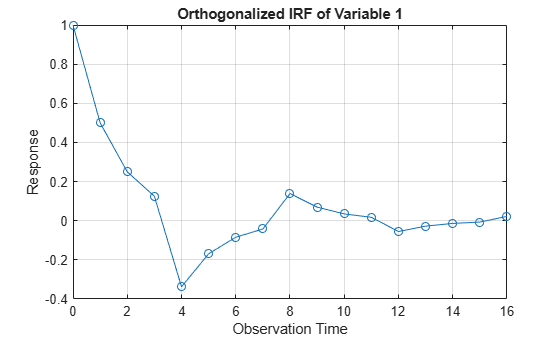Compare the IRFs.

```periods = (0:(numObs - 1))'; table(periods,irfIMPULSE,irfARMAIRF)```
```ans=17×3 table periods irfIMPULSE irfARMAIRF _______ __________ __________ 0 1 1 1 0.5 0.5 2 0.25 0.25 3 0.125 0.125 4 -0.3375 -0.3375 5 -0.16875 -0.16875 6 -0.084375 -0.084375 7 -0.042188 -0.042188 8 0.13891 0.13891 9 0.069453 0.069453 10 0.034727 0.034727 11 0.017363 0.017363 12 -0.055318 -0.055318 13 -0.027659 -0.027659 14 -0.01383 -0.01383 15 -0.0069148 -0.0069148 ⋮ ```

The IRFs returned by the two functions appear equivalent.

### More About the Impulse Response Function

Consider the general linear model of a univariate time series yt

`$a\left(L\right){y}_{t}=c+{x}_{t}\beta +b\left(L\right){\epsilon }_{t},$`

where:

• {εt} is a sequence of uncorrelated, identically distributed random variables with standard deviation σ.

• a(L) is an AR lag operator polynomial.

• c is the model constant.

• xtβ is the exogenous regression component. xt is a row vector of observations of the exogenous variables at time t, and β is the corresponding column vector of regression coefficients.

• b(L) is an MA lag operator polynomial.

Assuming a(L) is nonzero, a succinct representation of the model is

`${y}_{t}={m}_{t}+\psi \left(L\right){\epsilon }_{t},$`

where:

• $\psi \left(L\right)=a{\left(L\right)}^{-1}b\left(L\right)$ is the infinite-degree MA lag operator polynomial ${\psi }_{0}+{\psi }_{1}L+{\psi }_{2}{L}^{2}+\dots$ with scalar coefficients ψj, j = 0,1,2,… and ψ0 = 1.

• mt is the deterministic, innovation-free conditional mean of the process at time t.

The impulse response function (IRF) is the dynamic response of the system to a single impulse (innovation shock). The IRF measures the change to the response j periods in the future due to a change in the innovation at time t, for j = 0,1,2,…. Symbolically, the IRF at period j is

`$\frac{\partial {y}_{t+j}}{\partial {\epsilon }_{t}}={\psi }_{j}.$`

The sequence of dynamic multipliers , ψ0, ψ1, ψ2,..., measures the sensitivity of the process to a purely transitory change in the innovation process, with past responses and future innovations set to 0. Because the partial derivative is taken with respect to the innovation, the presence of deterministic terms in the model, such as the constant and the exogenous regression component, has no effect on the impulse responses.

Properties of the IRF determine characteristics of the process:

• If the sequence $\left\{{\psi }_{j}\right\}$ is absolutely summable, yt is a covariance-stationary stochastic process . For a stationary stochastic process, the impact on the process due to a change in εt is not permanent, and the effect of the impulse decays to zero.

• Otherwise, the process yt is nonstationary, and a change in εt affects the process permanently.

Because innovations can be interpreted as one-step-ahead forecast errors, the impulse response is also known as the forecast error impulse response.

 Hamilton, James D. Time Series Analysis. Princeton, NJ: Princeton University Press, 1994.

 Wold, Herman. "A Study in the Analysis of Stationary Time Series." Journal of the Institute of Actuaries 70 (March 1939): 113–115. https://doi.org/10.1017/S0020268100011574.